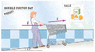wr1015
A shopper pushes a 7.3 kg shopping cart up a 13° incline, as shown in Figure 5-21. Find the horizontal force, F, needed to give the cart an acceleration of 1.63 m/s2.

ok, here is the formula to find horizontal force:

sum Fx= F1x +F2x = F1 + F2cos(theta), which also =mAx

if there are no forces given, how do i calculate F2cos(13degrees) and F2sin(13degrees)?

#### Attachments

•05-21.gif
18.6 KB · Views: 442
Last edited:

Staff Emeritus
Gold Member
wr1015 said:
if there are no forces given, how do i calculate F2cos(13degrees) and F2sin(13degrees)?

wr1015
Tom Mattson said:

my answer is supposed to be in Newtons, but my question is how to calculate acceleration in the x direction??

Staff Emeritus
Gold Member
Duh, I didn't read the problem statement very carefully. :tongue: Yes, you will get an answer in Newtons.

Instead of resorting to a canned formula, you should draw a free body diagram and write down equations for the sum of the forces in the x and y directions. You've got the normal force, the applied force, and gravity.

wr1015
Tom Mattson said:
Duh, I didn't read the problem statement very carefully. :tongue: Yes, you will get an answer in Newtons.

Instead of resorting to a canned formula, you should draw a free body diagram and write down equations for the sum of the forces in the x and y directions. You've got the normal force, the applied force, and gravity.

ok so normal force = mass of cart x acceleration of gravity: F=(7.3kg)(9.81 m/s2) = 71.613N, what's the applied force?

Staff Emeritus